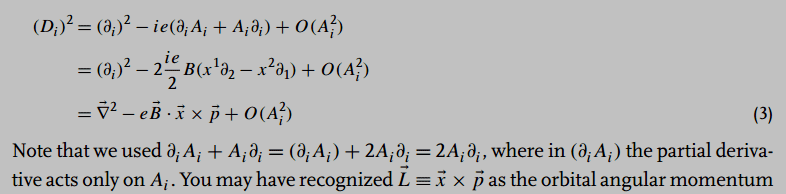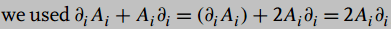# Zee, QFT Nut, III.6, p. 194

• A
• Lapidus

#### Lapidus

..where can be found:What in the whole wide world does Zee mean with??

Thank you

I guess that ##(\partial_i A_i)##is another way to say div A, which probably vanishes in the gauge Zee is considering. The bracket probably means that the action of the partial derivative is restricted to A.

•Lapidus
But on what else could the partial derivative act on than on A?

On what does it act in ##A_i \partial_i##? I mean, you are considering a momentum operator, so it will act on some wavefunction, besides A.

•Lapidus
A better way to write this would be
$$(\partial_i A_i + A_i \partial_i) X = (\partial_i A_i)X + 2 A_i \partial_i X = 2 A_i \partial_i X$$
for some wave function X and ignoring indices there. Just the product rule applied to ##\partial_i A_i X##

Last edited:
•Lapidus
Got it! Thanks DrDu and mfb!

This book by Zee, although apparently written in a quite colloquial way, confuses (at least me) more than it helps. This is an example. Instead of writing the simple matter in a simple way as mfb did in #5 he only gives some short-hand notation, which is more confusing than helpful :-(.

Well it's so and so; it seems like a bigger generalization of the derivation of $xp -px = i \hbar$. Without having to write $(xp - px ) f(x) = i \hbar f(x)$.
Although I think similar expressions were in Griffith's Intro to Electrodynamics.
$$(\partial_i A_i + A_i \partial_i) X = (\partial_i A_i) + 2 A_i \partial_i X = 2 A_i \partial_i X$$
Just to point out a small misprint (mfb just forgot): there is an X missing in the middle expression$$(\partial_i A_i + A_i \partial_i) X = (\partial_i A_i) X + 2 A_i \partial_i X = 2 A_i \partial_i X$$
•